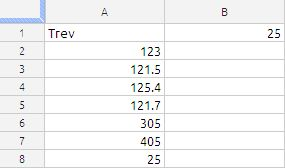## OFFSET FUNCTION

The OFFSET function returns a range of cells that is the specified number of rows and columns away from the specified base cell.

OFFSET(reference, rows, columns, height, width)

reference:  The address of the cell from which the offsets are measured.

rows: The number of rows from the reference cell to the target cell. rows is a number value. 0 means the target cell is in the same row as the base cell. A negative number means the target is in a row above the reference.

columns: The number of columns from the reference cell to the target cell. columns is a number value. 0 means the target cell is in the same column as the refernce cell. A negative number means the target is in a column to the left of the base.

height:  An optional value specifying the number of rows to return starting with the offset location. height  is a number value.

width:  An optional value specifying the number of columns to return starting with the offset location. width is a number value.

Usage Notes

OFFSET can return an array for use with another function. For example, assume you have entered into A1, A2, and A3, the base cell, the number of rows, and the number of columns, respectively, that you wish to have summed. The sum could be found using =SUM(OFFSET(INDIRECT(A1),0,0,A2,A3)).

Hello, I am a total spreadsheet n00b and I am banging my head on this problem: I have a huge amount of data I need to sum up. Every column has a few thousands rows, and I want to sum them up 16 by 16 in another cell. So, for example: A1:A16 summed in B1, A17:A32 summed in B2, and so on. Is there a clever and quick way of doing this, without manually changing a formula every time but just using one formula? I have tens of these columns to sum up, and it’s driving me kinda crazy, I tried searching for something similar to no avail.

the link to the blog is

the solution suggested by me in cell b1 is as follows:

=SUM(OFFSET(\$A\$1,(ROW()-1)*16,,16))

and drag it down

we have taken the first argument reference as cell A1 as absolute reference.

In the second argument is rows we have intelligently put the Row() function, this function will first evaluate the row() function since we are in cell B1 the row function will return 1 We are subtracting 1 from row() function which will give us the value 0 and it is multiplied by 16 and it will result in 0 that means the rows offset is 0 for the first cell indirectly we are setting the cell start the reference cell to A1.

When we drag the formula to cell B2 the row() function gets the value of 2 and subtracting 1 from that will give us the value 1 and multiplying this with 16 will give the reference cell as A17.

The third argument is Columns which we have ignored because there is no column offset the in the question

The fourth argument is height  we have assumed the value 16, since in the question he want to total of sum of every 16 rows

The fifth argument is left since it is a optional one.

If we try to solve the same problem using the other formulas the logic will be complicated.

## HOW TO RETRIEVE THE LAST VALUE IN A COLUMN

Suppose the data is laid as shown in the below imageThe formula in cell B1 is

=INDEX(A:A,COUNTA(A:A))

this will retrieve the value in the last cell of column A.

but the above formula will fail if the there are blank in the column A this can modified as follows:the formula in cell B1 is

=INDEX(A:A,MAX(ROW(A:A)*(A:A<>””)))

so if there are blanks in between data the above formula is written to ignore those black.